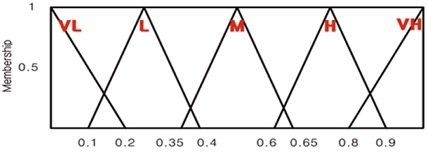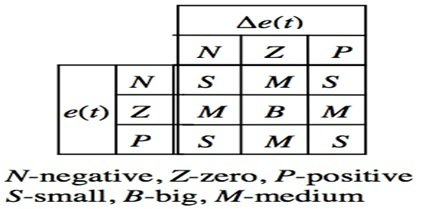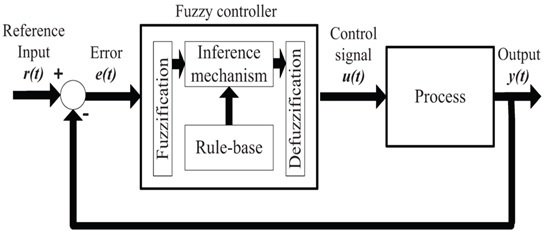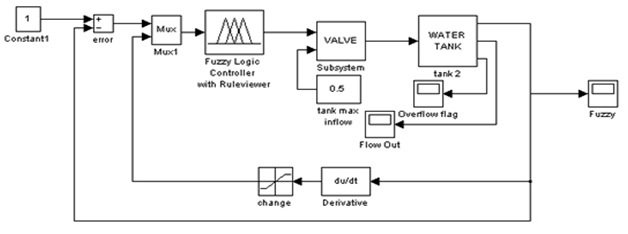# Fuzzy Logic System: How fuzzy logic control system works?

Fuzzy Logic Control System: What is Fuzzy Logic System?Fuzzy logic is a many valued logic in which truth values of variables may be any real number between 0 and 1. This logic is employed for obtaining the partial truth between true and false values. If we compare it with Boolean logic then in Boolean logic there are only two integer values 0 and 1 but in fuzzy logic system linguistic variables are used means non-numeric values such as age temperature etc. with their relative degrees and these degrees are managed by membership function. Which are shown in figure 1Figure 1 Fuzzy Logic Membership Functions

This figure has five membership functions very low (VL), low (L), medium(M), high (H) and very high (VH) and these membership functions have different values range such as (0.1,0.4), (0.35,.0.65,), (0.6,0.9) shown horizontally in figure 1. Similarly, these membership functions have two degrees such as 0.5 and 1 shown vertically in figure 1. So, the main difference between fuzzy logic and Boolean logic is that, Boolean logic has only two values 0 and 1 but fuzzy logic has many value between 0 and 1 such as 0.1,0.2, and 0.3 etc.

### How the Fuzzy Logic System Invented?

In 1960, Lotfi A Zadeh who was the professor in University of California at Berkley and he had well-known name in the field of development system theories at that time, feels that the traditional system analysis techniques are too precise for solving complex real-world problems. Then he had proposed an idea with grade membership function which had become the backbone of fuzzy set theory in 1964 and this idea was published in 1965 as a fuzzy set theory as well as the fuzzy logic technology was invented. Similarly, in 1974 the first fuzzy logic controller was developed in United Kingdom by S. Assilian and E.H Mamdani for controlling steam generator. After that, so many important industrial applications were developed by Japan in 1980 which were successfully launched in whole world. Then after eight-year constant research with development efforts, Yasunobu and his colleagues put a fuzzy logic control system on an automatic train in Sendai city subway system in 1987. So, this was the brief history about fuzzy logic invention.

### How Fuzzy Logic System Works?

The fuzzy logic system works based on fuzzy rules which are settled by fuzzy logic controller designer. Fuzzy logic controller has two inputs such as error (E) and change in error (DE) shown in figure 2

Figure 2 Fuzzy Logic System RulesThese fuzzy rules are put into fuzzy logic controller in this way,

If error is negative and change in error is also negative then output would be small,

If error is negative and change in error is zero then output would be medium,

If error is negative and change in error is positive then output would be small,

If error is zero and change in error is negative then output would be medium,

If error is zero and change in error is also zero then output would be big,

If error is zero and change in error is positive then output would be medium,

Similarly,

If error is positive and change in error is negative then output would be small,

If error is positive and change in error is zero then output would be medium,

If error is positive and change in error is also positive then output would be small,

These are the main fuzzy rules which are developed by fuzzy logic controller designer besides these rules the designer could also set further fuzzy rules for obtaining more precise output. The designer only sets these rules and based on these rules controller automatically sets the output of fuzzy logic controller.

### Fuzzy Logic Control System

A simple fuzzy logic control system is shown is figure 3Figure 3 Fuzzy Logic Control System

The fuzzy logic control system consists of two inputs error and change in error, error is obtained by comparing the reference input signal with output signal. This error is checked with respect to time that is called change in error and these are the basically two input of fuzzy logic controller. The fuzzy logic controller consists of three components fuzzification, inference mechanism and DE fuzzification which are explained in detail in below paragraph. When these inputs are given to fuzzy logic controller then fuzzy logic controller decided what would be the output of this controller using fuzzy rules which are settled by fuzzy controller designer. Similarly, the fuzzy logic controller output is given to output motor or machine after processing mechanism.

### Components of Fuzzy Logic Control System

The fuzzy logic control system consists of three main components,

Fuzzification: Fuzzification component consists of two further components that is called membership function and labels. Fuzzy logic controller converts input data or variable data into fuzzy membership function according to user defined chart such as temperature is too cold, motor speed is too low and assign the grade of this data value from 0 to 1 shown in figure 1. Different shapes could be used for membership function such as S, A, π and Z. A shape of membership function with labels such as low, medium and very low is shown is figure 1.

Inference Mechanism: Inference mechanism component of fuzzy logic control system consists of fuzzy rules which are settled by controller designer shown in figure 2. Based on these fuzzy rules, controller decided the output of fuzzy logic controller. This is the main intelligent control of this system.

DE fuzzification: DE fuzzification component of fuzzy logic converts the fuzzy data values into real life data values after examining the fuzzy rules but these real-life data values depend upon the DE fuzzification method. Different methods are used for DE fuzzification process such center of gravity (SOG), weighted average method, mean of maxima (MOM) and smallest of maxima (SOM). Each method has different advantages and disadvantages. These methods are set by the controller designer.

Example of Fuzzy Logic Controller with Explanation:

Using fuzzy logic controller, we can easily control the water level or oil level of any tank and here we would be explained the fuzzy logic controller by controlling the water level of any tank. Water level control figure is shown below.Figure 4 Water Level Control Using Fuzzy Logic Controller

For controlling the water level of any tank first the designer set the fuzzy rules according to figure 2 means at this point of water the valve is open and at this point the valve is closed. For this purpose, sensors are installed at water tank for checking the water level, these sensors sense the water level constantly and gives the input signal to fuzzy logic controller. Fuzzy logic controller also has the reference signal means the water level should be stand at this point. This reference signal is compared with sensor signal and error is generate and this error is checked with respect to time means it is increasing or decreasing these are basically the two input of fuzzy logic controller. Then fuzzy logic controller on or off the motor which relates to water valve means when water level is below the set value then open the water valve through motor and when water level is below the set value then close the water valve.